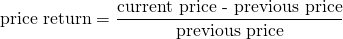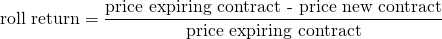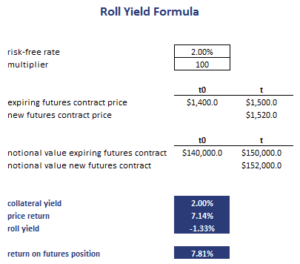# Roll Yield

The futures Roll Yield (RY) formula, or roll return, is an important concept that investors should understand well before trading futures. This is because the RY is a kind of yield that investors will not encounter when investing in stocks and bonds. The RY is part of the overall return that an investor earns when buying a futures contract. Thus, we also discuss the two other components before we turn to the roll yield formula.

## Futures return components

There are three components to a futures return. The first component is the collateral return or collateral yield. Investors have to provide collateral, i.e. post margin, when opening a futures position. The money or securities they provide as collateral will generally yield a positive return. This is the collateral yield. It depends on the margin posted. In the case the position is fully collateralized, we can approximate the collateral yield as the notional value times the risk-free rate.

The second component is the price return or spot yield. This is simply the futures return that is a result of the fact that the spot price of the underlying is changing over time. We can calculate the spot yield as## Roll yield definition

Finally, there is the roll return. Whether the roll return is positive or negative depends on the shape of the futures curve. If the futures curve is in contango, futures prices for more distant contracts are higher. In that case the RY is negative. When the futures market is in backwardation, the roll yield is positive. To see why this is the case, remember that the futures price always converges to the spot prices as the contract matures. Thus, if the futures curve is in backwardation, the spot price is above the futures price. Thus, the investor will earn a positive roll return simply by holding that contract.  The RY in contango is negative.

The formula to calculate the roll return is the following## Roll yield formula example

Let’s consider an example of how we can calculate the different components of the futures return. The following table implements such a simple example. The roll yield formula Excel spreadsheet can be downloaded below.## Summary

We discussed the roll yield meaning and how the roll yield can be calculated. To do so, we need to have the price of the expiring contract as well as the price of the new contract we roll into.

### Roll return calculator

Want to have an implementation in Excel? Download the Excel file: RY Formula